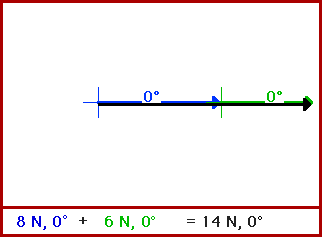## Vector Addition: 6 + 8 = ?

Most of us are accustomed to the following form of mathematics:

6 + 8 = 14.

6 + 8 = 10

and

6 + 8 = 2

and

6 + 8 = 5.

When we become students of physics and approach the task of adding vector quantities, we soon become aware of the fact that the addition of two vector quantities with magnitudes of 6 and 8 will not necessarily result in an answer of 14. The rules for adding vector quantities are different than the rules for adding two quantities arithmetically. Thus, vectors with magnitudes of 6 + 8 will not necessarily sum to 14.

Vectors are quantities which include a direction. As such, the addition of two or more vectors must take into account that the quantities being added have a directional characteristic. There are a number of methods for carrying out the addition of two (or more) vectors. The most common method is the head-to-tail method of vector addition. Using such a method, the first vector is drawn to scale in the appropriate direction. The second vector is then drawn such that its tail is positioned at the head (vector arrow) of the first vector. The sum of two such vectors is then represented by a third vector which stretches from the tail of the first vector to the head of the second vector. This third vector is known as the resultant - it is the result of adding the two vectors. The resultant is the vector sum of the two individual vectors. Of course, the actual magnitude and direction of the resultant is dependent upon the direction which the two individual vectors have.

This principle of the head-to-tail addition of vectors is illustrated in the animation below. In each frame of the animation, a vector with magnitude of 6 (in green) is added to a vector with magnitude of 8 (in blue). The resultant is depicted by a black vector which stretches from the tail of the first vector (8 units) to the head of the second vector (6 units).As can be seen from this animation, 8 + 6 could be equal to 14, but only if the two vectors are directed in the same direction. All that can be said for certain is that 8 + 6 can add up to a vector with a maximum magnitude of 14 and a minimum magnitude of 2. The maximum is obtained when the two vectors are directed in the same direction. The minimum s obtained when the two vectors are directed in the opposite direction.

For more information on physical descriptions of motion, visit The Physics Classroom Tutorial. Detailed information is available there on the following topics:

Vectors and Direction